## Mathematics Questions and Answers - Form 2 Term 3 Opener Exams 2022

INSTRUCTION TO STUDENTS:

• This paper consists of two Sections; Section I and Section II.
• Answer ALL the questions in Section I and only five questions from Section II.
• Show all the steps in your calculation, giving your answer at each stage in the spaces provided below each question.
• Marks may be given for correct working even if the answer is wrong.
• KNEC Mathematical tables may be used, except where stated otherwise.
• Students should answer the questions in English.

SECTION I (50 MARKS)

Answer ALL the questions in this section in the spaces provided.

1. Evaluate      (3 Marks)
−12 ÷ 3 × 4 −( −15)
−5 ×6 ÷ 2+( −5)
2. Evaluate without using a calculator. (3 Marks)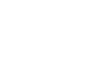3. Use logarithms to evaluate. (4 Marks)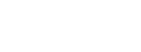4. Find the equation of the L1 in the form y=mx+c which is perpendicular to the line 3y + 2x = 6 and passes through the point (−3, 4). ( 3mks)
5. The diagram below represents a prism of length 6cm whose cross-section is an equilateral triangle of sides 3cm. Draw a well labeled sketch of the net of the prism. (3 Marks)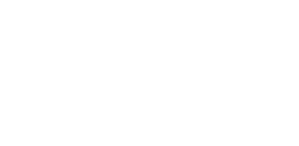6. The figure below represents a kite ABCD, AB = AD = 15 cm. The diagonals BD and AC intersect at O. AC = 30cm and AO = 12 cm. Find the area of the kite (3mks)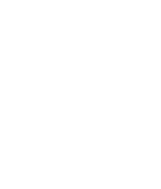7. Mrs. Musyoka has Sh. 700 in Sh. 50 notes and Sh. 100 notes only. If she has a total of 11 notes find how many notes she has of each denomination. (3mks)
8. In the figure below AD // BC. AC and BD intersect at E. Given that AE: EC = 1:5 and BD =12 cm, calculate the length of DE.    (3 marks)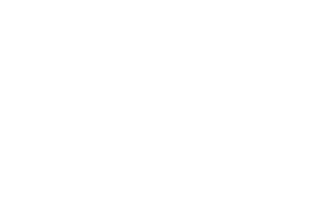9. Given that (3x−35) =cos (x+20) . Find (x+10)     (3mks)
10. Without using a mathematical tables or a calculator evaluate      (3mks)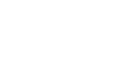11. Three bells ring at intervals of 9 minutes, 15 minutes and 21 minutes. The bells will next ring together at 11.00pm. Find the time the bells had last rung together. (3 Marks)
12. The surface areas of two similar bottles are 12cm2 and 108cm2 respectively. If the bigger one has a volume of 810cm3. Find the volume of the smaller one. (3 Marks)
13. In the figure below A′B′ is the image of AB under rotation. By construction, find and label the centre O of the rotation. Hence, determine the angle of the rotation.(4mks)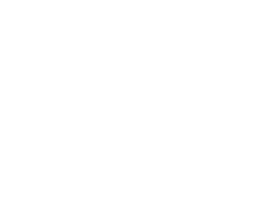14. Mr. Ombogo the principal of Chiga secondary would wish to cover the floor of the new administration block using the square tiles. The floor is a rectangle of sides 12.8m by 8.4m. Find the area of each of the largest tiles which can be used to fit exactly without breaking (3mks)
15. The size of an interior angle of a regular polygon is (3x)° while the exterior angle is (x+20)°. Find the number sides of the polygon (3 Marks)
16. In the figure below triangle ABO represents a part of a school badge. The badge has as symmetry of order 4 about O. Complete the figure to show the badge. (3mks)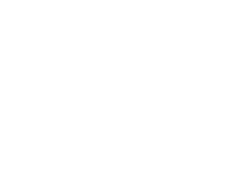SECTION II (50MKS)

Answer 5 questions only in this section

1. The vertices of quadrilateral OPQR are O (0, 0), P (2, 0), Q (4, 2) and R (0, 3). The vertices of its image under a rotation are O’ (1, -1), P'(1, -3) Q'(3, -5) an R'(4, -1).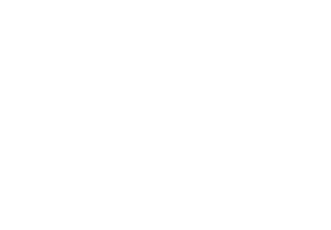1. On the grid provided, draw OPQR and its image O'P'Q'R' (2marks)
2. By construction, determine the centre and angle of rotation. (3marks)
3. On the same grid as (a) (i) above, draw O''P''Q''R'', the image of O'P'Q'R' under a reflection in the line y = x (3marks)
4. From the quadrilaterals drawn, state the pairs that are:
1. Directly congruent; (2marks)
2. Oppositely congruent (2marks)
2. A slaughter house bought a number of goats at Sh. 2000 each and a number of bulls at Sh. 15000 each. They paid a total of Sh. 190,000. If they bought twice as many goats and three bulls less, they would have saved Sh. 5000.
1. If the number of goats and bulls bought were x and y respectively, write down two simplified equations involving the above information. (2mks)
2. Solve the two equations above and hence find the number of each type of animals bought. (4mks)
3. The slaughter house sold all the animals at a profit of 25% per goat and 30% per bull. Determine the total profit they made. (4 Marks)
3.
1. In a safari rally drivers are to follow route ABCDA. B is 250km from A on a bearing of 075° from A. C is on a bearing of 110° from A and 280km from B. The bearing of C from D is 040° and a distance of 300km. By scale drawing show the position of the point A, B, C and D. (4mks)
2. Determine
1. The distance of A from C. (2mks)
2. The bearing of B from C. (1mk)
3. The bearing of A from D. (1mks)
4. The distance A from D (2mks)
4. A saleswoman is paid a commission of 20% on goods sold worth over Ksh 100,000.She is also paid a monthly salary of Ksh 12,000.In a certain month, she sold 360 handbags at Ksh 500 each.
1. Calculate the saleswoman’s earnings that month. (3 mks)
2. The following month, the saleswoman’s monthly salary was increased by 10%.Her to total earnings that month were Ksh17, 600.
Calculate the total amount of money received from the sales of handbags that month.(5mks)
3. The number of handbags sold that month. (2 mks)
5. Using a ruler and a pair of compasses only, draw a parallelogram ABCD, such that angle DAB = 75°. Length AB = 6.0cm and BC = 4.0cm.
From point D, drop a perpendicular to meet line AB at N. (7 Marks)
1. Measure length DN. (1 Mark)
2. Find the area of the parallelogram. (2 Marks)
6.
1. Two cubes of length 5cm and 7cm are melted and cast into a single cube.
Determine the:
1. Volume of the new cube (3mks)
2. Length of the new cube correct to 1 decimal place(2mks)
3. Surface area of the new cube (2mks)
2. Suppose that it was instead cast into a cylinder of radius 3.5 cm. what would the height be to the nearest cm? Take = 22/7 ,(3 mks)
7. The diagram below shows vertical telephone pole RS supported by wires SP and SQ pegged at points P and Q respectively on a level ground. Points P and Q are on the same straight line from the base R of the pole. The angles of elevation of S from P and Q are 33.9° and 48.2° respectively. Given that PR = 5 m, calculate: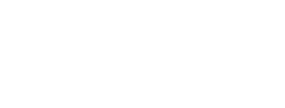1. The distance QR (4 marks)
2. The length of the wires SP and SQ (4 marks)
3. If the cost of the pole and labour is sh. 1600 and the cost of 1 meter of the wire is sh. 233. Find the total cost of the installation. (2 marks)
8. A glass in the form of a frustum of a cone, is represented by the diagram below. The glass contains water to a height of 9 cm. The bottom of the glass is a circle of radius 2 cm while the surface of the water is a circle of radius 6 cm.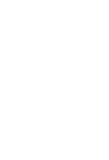1. Calculate the volume of the water in the glass. (3Mks)
2. When a special marble is submerged into the water in the glass, the water level rises by 1 cm.
Calculate :
1. the volume of the marble (4 marks)
2. the radius of the marble (3 marks)

### MARKING SCHEME

1. −4x4+15     √M1
−15 − 5
−1    √M1
−20
= 1/20 √A1
2. (17/7 − 11/6 ) x 6/5
2/3 × 9/4 − 8/7
Numerator = (102 − 77) x 6
42         5
= 25/426/5 = 5/7   √M1
Denominator = 3/2  − 8/7
= 21 − 16   √M1
14         14
5/7 ÷ 5/14 = 5/7  x 14/5
= 2 √A1
3. No.                log                 all logs √ M1
43.52          1.6387
0.08792       2.9441 +   √operations M1
0.5828
785.3           2.8950 –
3.6878÷4   √division by 4 M1
2.642 x 10-1 1.4220  Answer √ A1
4. 3y + 2x = 6
y = −2x/3 + 2
m1−2/3
m23/2  √B1
y − 43 = 3   √M1
x + 3     2
2( y − 4) = 3(x + 3)
2y − 8 = 3x + 9
2y = 3x + 17
Y = 3x/2  + 17/2   √A1
5.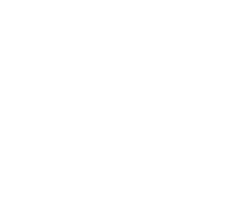6. BO = √(152 − 122) = 9 √B1
Area of kite = ½ x 30 x 9 x 2 √M1
= 270cm2√A1
7. Let sh.50 notes be x and sh.100 notes be y
X + y = 11 ....... (i)
50x + 100y = 700 Both equations √M1

5x + 10y = 70 ....... (ii)
X + y = 11 x 5
5x + 10y = 70 √Attempt to solve M1
5x + 5y   = 55
5y = 15
y = 3
x + 3 = 11
x = 8
sh50 = 8 notes
sh100 = 3 notes Both √A1
8. AE  = DE  = 1   √M1
EC     BE     5
DE       = 1   √M1
12-DE        5
5DE = 12 – DE
6DE = 12
DE = 2cm √A1
9. 3x – 35+x + 20 = 90 √M1
4x = 105
X = 26.25 √A1
Tan (26.25+10) = Tan36.25 = 0.7332 √B1
10.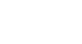=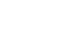M1
=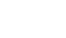M1
= 3 x 15
4
= 45/4 = 11¼ or 11.25 √A1
11.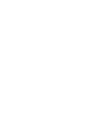LCM = 3 x 3 x 5 x 7 = 315 min √ determination of LCM
= 5h 15min M1
Last rung at 11.00p.m – 5h 15min √MI
= 5.45p.m √A1
12. ASF = 12/108 = 1/9
⇒ LSF = 1/3  √B1
V.S.F =(1/3)3 = 1/27
1/27  = V/810   √M1
⇒ V = 810 = 30cm3  √A1
27
13.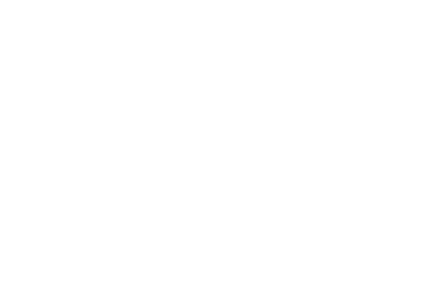14.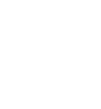GCD = 2x 2 x 2 x 5 = 40cm √A1
Area = 40 x 40 = 1600cm2√B1
15. 3x + x + 20 = 180°√M1
4x = 160°
x = 40°√A1
n = 360° = 9 √ B1
40°
16.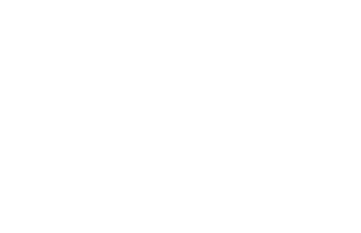17.
1.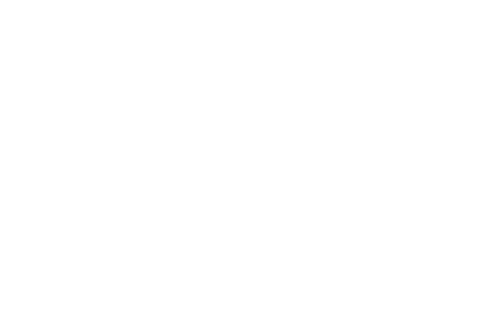2. Centre (0, −1)  ∠ of rotation = −90°
3.
4.
1. OPQR & O'P'Q'R'     ✓Both B2
2. O'P'Q'R' & O''P''Q''R''    ✓any 2 B2
OR
OPQR & O''P''Q''R''
18.
1. 2000x + 15000y = 190000
2x + 15y = 190 .....  (i) √B1
2 x 2000x+ (y−3) 15000 = 185000
4x + (y−3)15 = 185
4x + 5y−45 = 185
4x + 5y = 230 .........(ii) √B1
2. 2x + 15y = 190
4x + 5y = 230
4x + 30y = 380 √attempt to solve M1
4x + 5y = 230
25y = 150
y = 6 √AI
4x + 5x6 = 230 √M1
4x = 200
X = 50 √A1
Goats = 50
Bulls = 6
3. Profit from goats = 25/100 x 2000x50 = sh25000 √M1
Profit from Bulls = 30/100 x 15000x6 = sh27000 √M1
Total profit = 25000 + 27000 √M1
= sh52,000√AI
19.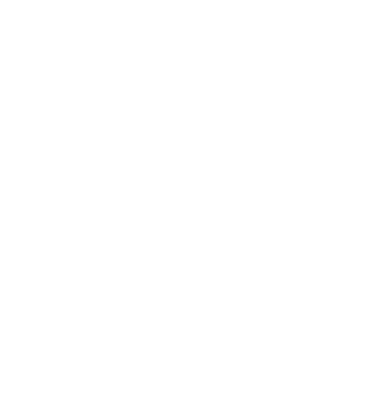20.
1. Handbag sales = 360 x 500 = 180000 √BI
Commission = 20/100 x 80,000 = sh16000 √BI
Total earnings = 12000 + 16000 = 28000 √BI
2. Sales woman salary = 110/100  x 12000 = sh13200 √BI
Commission = 17600 – 13200 = 4400 √MI
Money received from Handbag sales = 4400 x 100 + 100,000 √√ M2
20
= sh120,000√A1
3. No. of handbags = 120,000 √M1
500
= 240 √A1
21.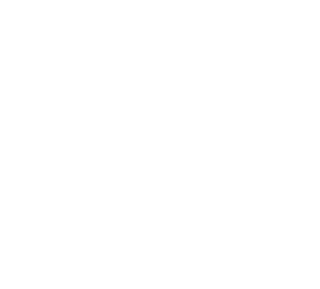1. DN = 3.9cm
2. Area = ½ × 6 × 3.9
= 11.7cm2
22.
1.
1. Vol = 53 + 73√ M1
= 125 + 343 √M1
= 468 √A1
2. L3 = 468
L = 3√468   √M1
= 7.764
≅ 7.8cm √A1
3. SA= 6a2
= 6(7.8)2
= 365.04cm2
2. 22/7 x 3.52 x h = 468 √M1
h = 468 x 7
22x3.52
468 x 7
22x12.25
= 12.16 √A1
≅12cm √B1
23.
1. Tan 33.9 = SR/5 ⇒ SR = 5 tan 33.9 √M1
Tan 48.2 =  SR  ⇒ SR = (5−x) tan 48.2 √M1
5−x
5 x 0.672 = 1.118(5−x) √M1
3.36 = 5.59 – 1.118x
1.118x = 2.23
x=  2.23  = 1.995 √A1
1.118
2. Cos 33.9 = 5/SP
SP =       5           5        √M1
Cos33.9     0.8300
= 6.024m
Cos 48.2 = 1.995  √M1
SQ
SQ =   1.995        =  1.995    √M1
Cos48.2         0.6665
= 2.993 √A1
3. Cost of wire = (6.024+2.993) x 233
= 9.017 X 233 = 2100.96
≅ 2101 √B1
Total cost = 2101 + 1600
= sh3701 √B1
24.
1. Volume of water =  6   = 2/x    M1
9+x
x = 4.5  M1
; volume
= 1/3x 3.142(62 x 13.5 − 23 x 4.5)
= 508.94 − 18.25 = 490.09  A1
2.
1. Volume of sphere   M1
Top radium r/14.5 = 2/4.5   r=6.440  A1
Volume = 1/3 x 3.142 (6.4442 x 14.5 − 62 x 13.5)   M1
= 121.6 = 121 11/15     A1
2. 4/3Лr3 = 121.6      M1
r3 = 121.6 x ¾x    M1
r = 3.073       A1
10Mrks

• ✔ To read offline at any time.
• ✔ To Print at your convenience
• ✔ Share Easily with Friends / Students